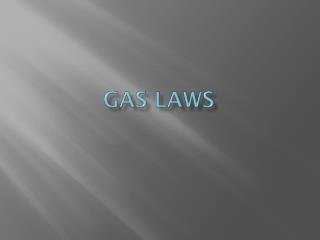Download PresentationGas Laws

# Gas Laws

Download Presentation## Gas Laws

- - - - - - - - - - - - - - - - - - - - - - - - - - - E N D - - - - - - - - - - - - - - - - - - - - - - - - - - -
##### Presentation Transcript

1. Gas Laws

2. Factors • Pressure • Volume • Moles • Temperature

3. Pressure • Common units • Atmospheres (atm) • mm Hg • Torr • kiloPascals (kPa) • Pounds per square inch (psi) • Bar or millibar (b or mb) • Influences • Weight of air or water above you • Force caused by gas particle collisions with the container wall

4. Standard Pressure • 1 atm • 760 torr • 760 mmHg • 101.325 kPa • 14.69 psi

5. Volume • Typically in Liters • mL = cc or cm3

6. Moles • Gas laws are independent of mass, but are dependent on moles, which represents number of particles • Exception is Graham’s Law

7. Temperature • Must use Kelvins • K = oC + 273.16, but we can get away with saying 273

8. Dynamic gas Laws • PV/nT = PV/nT • Cross out any factors that are either constant or unmentioned • Use this only if a factor is changing

9. Individual Laws • Boyles Law PV • Charles’ Law VT • Avogadro’s Law Vn • Gay-Lussac: PT

10. Static Gas LawAKA Ideal Gas Law • PV=nRT • Use when you have all factors but one and the system is not changing • R = ideal gas constant • Values for R: depends upon your unit of pressure • 0.08206 L atm/mol K • 62.36 L mmHg/mol K • 62.36 L torr / mol K • 8.314 L kPa/mol K

11. Dalton’s Law of Partial Pressures • Pressure Tot = S partial pressure • Mole fraction = pressure fraction

12. Dalton’s law in gas collection over water • Pressure Tot = P coll gas+P water vapor • Total Pressure = atmospheric pressure • Water vapor pressure can be looked up in a table by temperature • Pressure of dry gas = Atmospheric pressure – water vapor pressure

13. Dalton’s Law with mole fraction • Have a mixture of 2 mol He, 4 mol Kr, and 4 mol Ar. If the total pressure is 760 mmHg, what is the partial pressure of Ar? • Mole fraction of Ar = 4/(2+4+4) or .4 • Partial pressure of Ar = 760 x .4 • Partial pressure of Ar = 304 mmHg

14. Graham’s Law • Two gases in a mixture are at the same temperature • Same temp means same avg kinetic energy • ½ mv2= ½ mv2 • The lower mass will have the higher velocity • A lower mass gas will diffuse or effuse faster

15. Gas Stoichiometry • May have to use PV=nRT to find moles of known or unknown • Be careful with your phase symbols. ALL gases must be considered